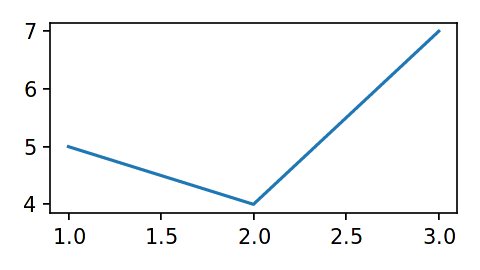# Backend: `matplotlib`

The `matplotlib` library is a - if not the - classic plotting package in the Python ecosystem. For further details, please consult the documentation of matplotlib. A good basic introduction can also be found in the Python Data Science Handbook (2016), chapter 4, by Jake VanderPlas. The author has made the manuscript freely available in the form of Jupyter notebooks on Github.

```from bewegung import Video

v = Video(width = 480, height = 270, seconds = 1.0)

@v.sequence()
class Foo:

@v.layer(
canvas = v.canvas(
backend = 'matplotlib',
facecolor = '#FFFFFFFF',
dpi = 150,
)
)
def bar(self, canvas): # a matplotlib figure object

ax = canvas.subplots()
ax.plot([1, 2, 3], [5, 4, 7])

return canvas

v.reset()
v.render_frame(v.time(0))
```Similar to `matplotlib.pyplot.figure`, the function call `v.canvas(backend = 'matplotlib')` accepts the following additional keyword arguments, among others (see documentation of matplotlib.pyplot.figure and matplotlib.figure.Figure):

• `dpi`, resolution or dots per inch. 300 by default.

• `figsize`, a tuple of width and height in inches. Width and height of the video by default, converted to inches based on the value of `dpi`.

• `width`, width in pixels. Mapped to `figsize` if provided together with `height`. Converted to inches based on the value of `dpi`.

• `height`, height in pixels. Mapped to `figsize` if provided together with `width`. Converted to inches based on the value of `dpi`.

• `tight_layout`, by default `True`.

• `facecolor`, a background color.

• `background_color`, mapped to `facecolor`. Accepts `bewegung.Color` objects.

• `managed`, a boolean, by default `True`. This value indicates whether the the `matplotlib.figure.Figure` object is “managed” by `bewegung`. If `True`, `bewegung` will close, i.e. destroy, a figure that is returned by a layer method.

Layer methods are expected to return `matplotlib.figure.Figure` objects.

Warning

By default, `bewegung` will “manage” `matplotlib.figure.Figure` objects for saving resources, i.e. the returned `matplotlib.figure.Figure` objects are automatically closed once returned. This can be avoided by setting `managed` to `False`.

## Accelerating `matplotlib`

Aside from its rich set of features, `matplotlib` is known for its mediocre performance. Not to be confused with `bewegung`’s backends, `matplotlib` also has different backends for rendering. Within `bewegung`, `matplotlib` is automatically configured to use mplcairo, “A (new) cairo backend for Matplotlib”. Compared to `matplotlib`’s own built-in backends, its output quality is significantly better while the rendering speed is also higher. Unfortunately, `mplcairo` is just “half” of the story of `matplotlib` performance.

Warning

If `mplcairo` can not be installed or is not present for whatever reason, `bewegung` will show a warning and fall back to `matplotlib`’s internal `cairo` backend.

In animation frameworks for `matplotlib`, such as the “official” matplotlib.animation sub-package, it is common practice to re-use and update existing figure and subplot / axes objects. This speeds up the rendering process considerably. This strategy is also supported by `bewegung`.

Warning

For optimal results, the suggested approach requires some deeper understanding of `matplotlib`’s facilities.

The following code illustrates the approach.

```from bewegung import Video

v = Video(width = 480, height = 270, seconds = 1.0)

@v.sequence()
class Foo:

def __init__(self):

self._fig = v.canvas(
backend = 'matplotlib',
facecolor = '#FFFFFFFF',
dpi = 150,
managed = False, # ensure that bewegung does not close figure
)() # calls the factory once, generates a single figure
self._ax = self._fig.subplots() # generates a single subplot

@v.layer() # no backend configuration required
def bar(self): # no canvas requested

self._ax.clear() # optional: update content directly instead
self._ax.plot([1, 2, 3], [5, 4, 7]) # draw new content or change old content

return self._fig

v.reset()
image0 = v.render_frame(v.time(0))
image1 = v.render_frame(v.time(1))
```

The less a figure changes, the faster the above code becomes. Depending on the degree of complexity and optimization, anything from a few percent to an order of magnitude of performance gain can be achieved.